moveit2 The MoveIt Motion Planning Framework for ROS 2.
kinematic_constraints::JointConstraint Class Reference

Class for handling single DOF joint constraints. More...

`#include <kinematic_constraint.h>`

Inheritance diagram for kinematic_constraints::JointConstraint: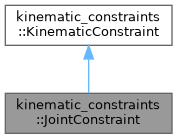[legend]
Collaboration diagram for kinematic_constraints::JointConstraint: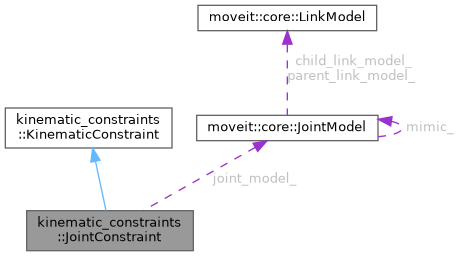[legend]

## Public Member Functions

JointConstraint (const moveit::core::RobotModelConstPtr &model)
Constructor. More...

bool configure (const moveit_msgs::msg::JointConstraint &jc)
Configure the constraint based on a moveit_msgs::msg::JointConstraint. More...

bool equal (const KinematicConstraint &other, double margin) const override
Check if two joint constraints are the same. More...

ConstraintEvaluationResult decide (const moveit::core::RobotState &state, bool verbose=false) const override
Decide whether the constraint is satisfied in the indicated state. More...

bool enabled () const override
This function returns true if this constraint is configured and able to decide whether states do meet the constraint or not. If this function returns false it means that decide() will always return true – there is no constraint to be checked. More...

void clear () override
Clear the stored constraint. More...

void print (std::ostream &out=std::cout) const override
Print the constraint data. More...

const moveit::core::JointModelgetJointModel () const
Get the joint model for which this constraint operates. More...

const std::string & getLocalVariableName () const
Gets the local variable name if this constraint was configured for a part of a multi-DOF joint. More...

const std::string & getJointVariableName () const
Gets the joint variable name, as known to the moveit::core::RobotModel. More...

int getJointVariableIndex () const

double getDesiredJointPosition () const
Gets the desired position component of the constraint. More...

double getJointToleranceAbove () const
Gets the upper tolerance component of the joint constraint. More...

double getJointToleranceBelow () const
Gets the lower tolerance component of the joint constraint. More...Public Member Functions inherited from kinematic_constraints::KinematicConstraint
KinematicConstraint (const moveit::core::RobotModelConstPtr &model)
Constructor. More...

virtual ~KinematicConstraint ()

ConstraintType getType () const
Get the type of constraint. More...

double getConstraintWeight () const
The weight of a constraint is a multiplicative factor associated to the distance computed by the decide() function. More...

const moveit::core::RobotModelConstPtr & getRobotModel () const

## Protected Attributes

const moveit::core::JointModeljoint_model_
The joint from the kinematic model for this constraint. More...

bool joint_is_continuous_
Whether or not the joint is continuous. More...

std::string local_variable_name_
The local variable name for a multi DOF joint, if any. More...

std::string joint_variable_name_
The joint variable name. More...

int joint_variable_index_
The index of the joint variable name in the full robot state. More...

double joint_position_

double joint_tolerance_above_

double joint_tolerance_below_
Position and tolerance values. More...Protected Attributes inherited from kinematic_constraints::KinematicConstraint
ConstraintType type_
The type of the constraint. More...

moveit::core::RobotModelConstPtr robot_model_
The kinematic model associated with this constraint. More...

double constraint_weight_
The weight of a constraint is a multiplicative factor associated to the distance computed by the decide() function
More...Public Types inherited from kinematic_constraints::KinematicConstraint
enum  ConstraintType {
UNKNOWN_CONSTRAINT , JOINT_CONSTRAINT , POSITION_CONSTRAINT , ORIENTATION_CONSTRAINT ,
VISIBILITY_CONSTRAINT
}
Enum for representing a constraint. More...

## Detailed Description

Class for handling single DOF joint constraints.

This class handles single DOF constraints expressed as a tolerance above and below a target position. Multi-DOF joints can be accommodated by using local name formulations - i.e. for a planar joint specifying a constraint in terms of "planar_joint_name"/x.

Continuous revolute single DOF joints will be evaluated based on wrapping around 3.14 and -3.14. Tolerances above and below will be evaluating over the wrap. For instance, if the constraint value is 3.14 and the tolerance above is .04, a value of -3.14 is in bounds, as is a value of -3.12. -3.1 is out of bounds. Similarly, if the value of the constraint is -3.14, the tolerance above is .04, and the tolerance below is .02 then -3.1 is a valid value, as is 3.14; 3.1 is out of bounds.

Type will be JOINT_CONSTRAINT.

Definition at line 202 of file kinematic_constraint.h.

## ◆ JointConstraint()

 kinematic_constraints::JointConstraint::JointConstraint ( const moveit::core::RobotModelConstPtr & model )
inline

Constructor.

Parameters
 [in] model The kinematic model used for constraint evaluation

Definition at line 210 of file kinematic_constraint.h.

## ◆ clear()

 void kinematic_constraints::JointConstraint::clear ( )
overridevirtual

Clear the stored constraint.

Implements kinematic_constraints::KinematicConstraint.

Definition at line 327 of file kinematic_constraint.cpp.

Here is the caller graph for this function: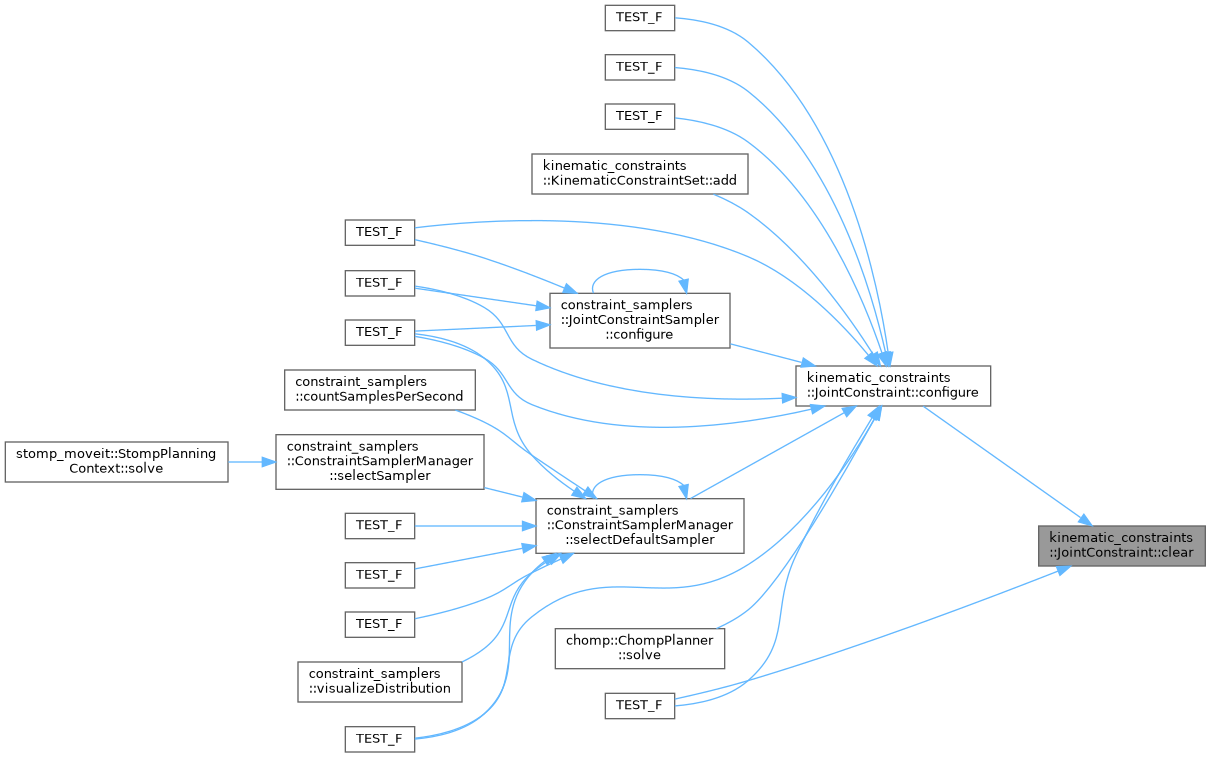## ◆ configure()

 bool kinematic_constraints::JointConstraint::configure ( const moveit_msgs::msg::JointConstraint & jc )

Configure the constraint based on a moveit_msgs::msg::JointConstraint.

For the configure command to be successful, the joint must exist in the kinematic model, the joint must not be a multi-DOF joint (for these joints, local variables should be used), and the tolerance values must be positive.

Parameters
 [in] jc JointConstraint for configuration
Returns
True if constraint can be configured from jc

Definition at line 134 of file kinematic_constraint.cpp.

Here is the call graph for this function: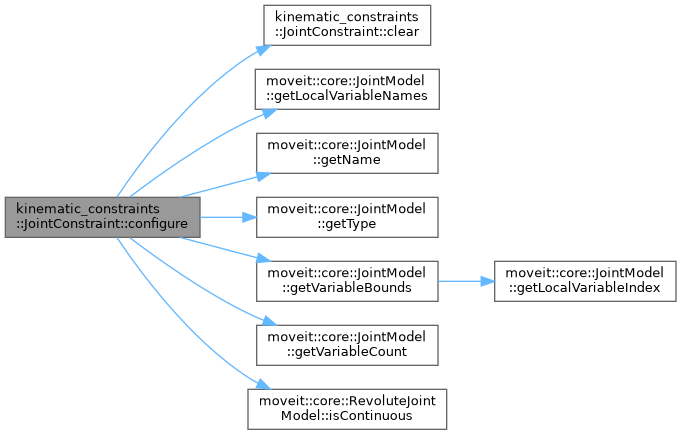Here is the caller graph for this function: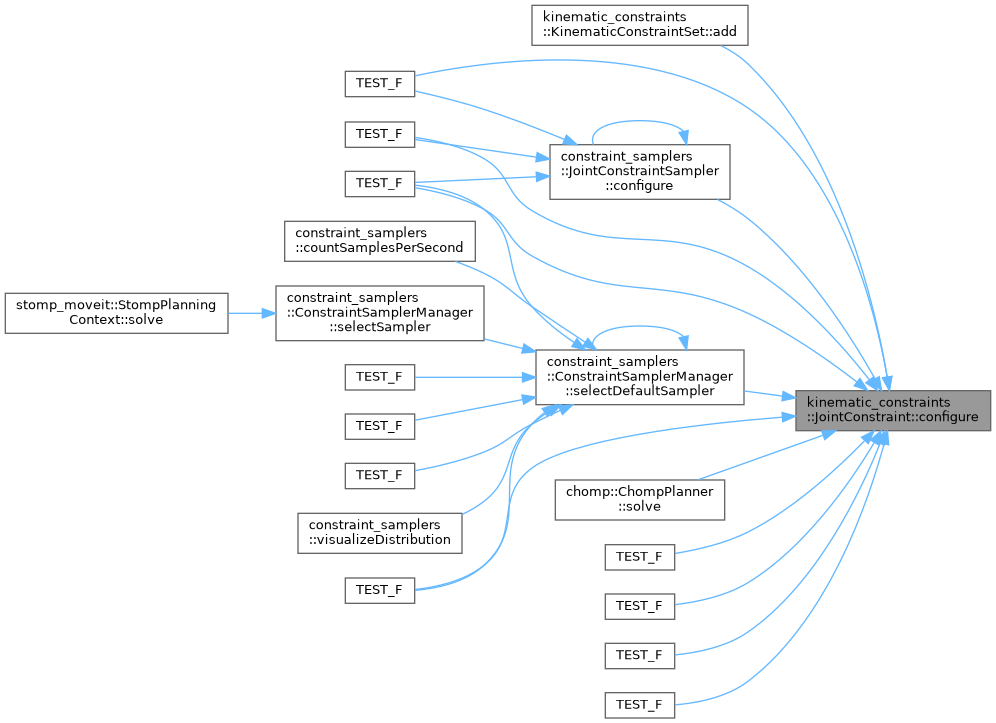## ◆ decide()

 ConstraintEvaluationResult kinematic_constraints::JointConstraint::decide ( const moveit::core::RobotState & state, bool verbose = `false` ) const
overridevirtual

Decide whether the constraint is satisfied in the indicated state.

Parameters
 [in] state The kinematic state used for evaluation [in] verbose Whether or not to print output
Returns

Implements kinematic_constraints::KinematicConstraint.

Definition at line 283 of file kinematic_constraint.cpp.

Here is the call graph for this function: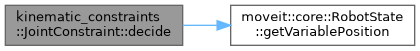Here is the caller graph for this function: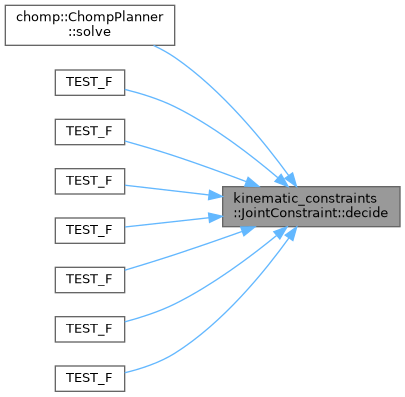## ◆ enabled()

 bool kinematic_constraints::JointConstraint::enabled ( ) const
overridevirtual

This function returns true if this constraint is configured and able to decide whether states do meet the constraint or not. If this function returns false it means that decide() will always return true – there is no constraint to be checked.

Implements kinematic_constraints::KinematicConstraint.

Definition at line 322 of file kinematic_constraint.cpp.

Here is the caller graph for this function: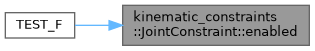## ◆ equal()

 bool kinematic_constraints::JointConstraint::equal ( const KinematicConstraint & other, double margin ) const
overridevirtual

Check if two joint constraints are the same.

This means that the types are the same, the subject of the constraint is the same, and all values associated with the constraint are within a margin. The other constraint must also be enabled. For this to be true of joint constraints, they must act on the same joint, and the position and tolerance values must be within the margins.

Parameters
 [in] other The other constraint to test [in] margin The margin to apply to all values associated with constraint
Returns
True if equal, otherwise false

Implements kinematic_constraints::KinematicConstraint.

Definition at line 269 of file kinematic_constraint.cpp.

Here is the call graph for this function: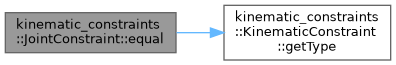Here is the caller graph for this function: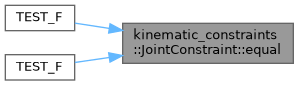## ◆ getDesiredJointPosition()

 double kinematic_constraints::JointConstraint::getDesiredJointPosition ( ) const
inline

Gets the desired position component of the constraint.

Returns
The desired joint position

Definition at line 298 of file kinematic_constraint.h.

## ◆ getJointModel()

 const moveit::core::JointModel* kinematic_constraints::JointConstraint::getJointModel ( ) const
inline

Get the joint model for which this constraint operates.

Returns
The relevant joint model if enabled, and otherwise nullptr

Definition at line 258 of file kinematic_constraint.h.

## ◆ getJointToleranceAbove()

 double kinematic_constraints::JointConstraint::getJointToleranceAbove ( ) const
inline

Gets the upper tolerance component of the joint constraint.

Returns
The above joint tolerance

Definition at line 309 of file kinematic_constraint.h.

## ◆ getJointToleranceBelow()

 double kinematic_constraints::JointConstraint::getJointToleranceBelow ( ) const
inline

Gets the lower tolerance component of the joint constraint.

Returns
The below joint tolerance

Definition at line 320 of file kinematic_constraint.h.

## ◆ getJointVariableIndex()

 int kinematic_constraints::JointConstraint::getJointVariableIndex ( ) const
inline

Definition at line 287 of file kinematic_constraint.h.

## ◆ getJointVariableName()

 const std::string& kinematic_constraints::JointConstraint::getJointVariableName ( ) const
inline

Gets the joint variable name, as known to the moveit::core::RobotModel.

This will include the local variable name if a variable of a multi-DOF joint is constrained.

Returns
The joint variable name

Definition at line 282 of file kinematic_constraint.h.

Here is the caller graph for this function:## ◆ getLocalVariableName()

 const std::string& kinematic_constraints::JointConstraint::getLocalVariableName ( ) const
inline

Gets the local variable name if this constraint was configured for a part of a multi-DOF joint.

Returns
The component of the joint name after the slash, or the empty string if there is no local variable name

Definition at line 270 of file kinematic_constraint.h.

## ◆ print()

 void kinematic_constraints::JointConstraint::print ( std::ostream & = `std::cout` ) const
overridevirtual

Print the constraint data.

Parameters
 [in] out The file descriptor for printing

Reimplemented from kinematic_constraints::KinematicConstraint.

Definition at line 337 of file kinematic_constraint.cpp.

## ◆ joint_is_continuous_

 bool kinematic_constraints::JointConstraint::joint_is_continuous_
protected

Whether or not the joint is continuous.

Definition at line 327 of file kinematic_constraint.h.

## ◆ joint_model_

 const moveit::core::JointModel* kinematic_constraints::JointConstraint::joint_model_
protected

The joint from the kinematic model for this constraint.

Definition at line 326 of file kinematic_constraint.h.

## ◆ joint_position_

 double kinematic_constraints::JointConstraint::joint_position_
protected

Definition at line 331 of file kinematic_constraint.h.

## ◆ joint_tolerance_above_

 double kinematic_constraints::JointConstraint::joint_tolerance_above_
protected

Definition at line 331 of file kinematic_constraint.h.

## ◆ joint_tolerance_below_

 double kinematic_constraints::JointConstraint::joint_tolerance_below_
protected

Position and tolerance values.

Definition at line 331 of file kinematic_constraint.h.

## ◆ joint_variable_index_

 int kinematic_constraints::JointConstraint::joint_variable_index_
protected

The index of the joint variable name in the full robot state.

Definition at line 330 of file kinematic_constraint.h.

## ◆ joint_variable_name_

 std::string kinematic_constraints::JointConstraint::joint_variable_name_
protected

The joint variable name.

Definition at line 329 of file kinematic_constraint.h.

## ◆ local_variable_name_

 std::string kinematic_constraints::JointConstraint::local_variable_name_
protected

The local variable name for a multi DOF joint, if any.

Definition at line 328 of file kinematic_constraint.h.

The documentation for this class was generated from the following files: